#Data Structure_树

0
0
01. 云栖社区>
2. 博客>
3. 正文

# 线段树Segment Tree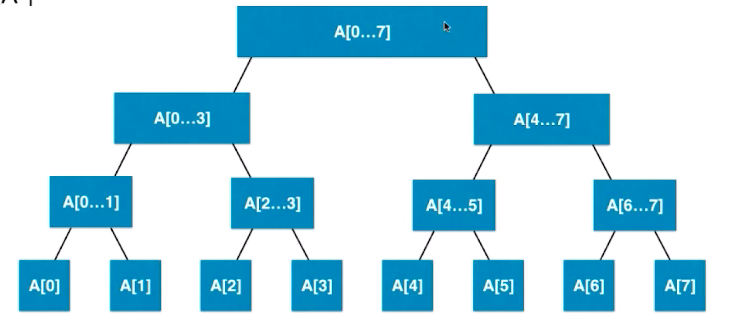，那么就需要2n个空间来存储。如果不是，那么就需要4n个，因为多出的那一个需要一整行取填补它。

#### Create

``````    private int leftChild(int index) {
return 2 * index + 1;
}

private int rightChild(int index) {
return 2 * index + 2;
}
``````

``````public class SegmentTree<E> {
private E[] data;
private E[] tree;
private Merger<E> merger;

public SegmentTree(E[] arr, Merger<E> merger) {
this.merger = merger;
data = (E[]) new Object[arr.length];
for (int i = 0; i < arr.length; i++) {
data[i] = arr[i];
}

tree = (E[]) new Object[4 * arr.length];
buildSegmentTree(0, 0, data.length - 1);
}
``````

data是传进来的数据，tree是树的数据merger是操作。还是递归，因为树这种数据结构用起递归是天然的方便。参数要有两个主要的参数，左边和右边的边界，其实按照上面的图就是中分。当l >= r的时候就是递归的最终条件，这个时候直接相等即可，否则就递归构建。

``````    private void buildSegmentTree(int treeIndex, int l, int r) {
if (l >= r) {
tree[treeIndex] = data[l];
return;
} else {
int leftTreeIndex = leftChild(treeIndex);
int rightTreeIndex = rightChild(treeIndex);
int mid = l + (r - l) / 2;
buildSegmentTree(leftTreeIndex, l, mid);
buildSegmentTree(rightTreeIndex, mid + 1, r);
tree[treeIndex] = merger.merger(tree[leftTreeIndex], tree[rightTreeIndex]);
}
}
``````

merger是一个接口，这是因为如果把这个功能写死了，那么线段树的功能就死了。比如求和，如果写死了那么这个树就只能求和。而如果加上了接口，最小值最大值也是可以的。线段树其实也是一种空间换时间的做法。

``````    public static void main(String[] args) {
Integer[] arr = {1, 2, 3, 4, 5, 6, 7, 8, 9, 10};
SegmentTree<Integer> segmentTree = new SegmentTree<Integer>(arr, (a, b) -> a > b?a:b);
segmentTree.show();
}
``````

#### Query

``````ry(int queryL, int queryR) {
if (queryL < 0 || queryL >= data.length || queryR < 0 || queryR >= data.length || queryL > queryR) {
throw new IllegalArgumentException("permater is illgel!");
}
return query(0, 0, data.length - 1, queryL, queryR);
}

private E query(int treeIndex, int l, int r, int queryL, int queryR) {
if (l == queryL && r == queryR) {
return tree[treeIndex];
}
int mid = l + (r - l) / 2;
int left = leftChild(treeIndex);
int right = rightChild(treeIndex);
if (queryL >= mid + 1) {
return query(right, mid + 1, r, queryL, queryR);
} else if (queryR <= mid) {
return query(left, l, mid, queryL, queryR);
} else {
return merger.merger(query(left, l, mid, queryL, mid), query(right, mid + 1, r, mid + 1, queryR));
}
}

``````

#### Leecode303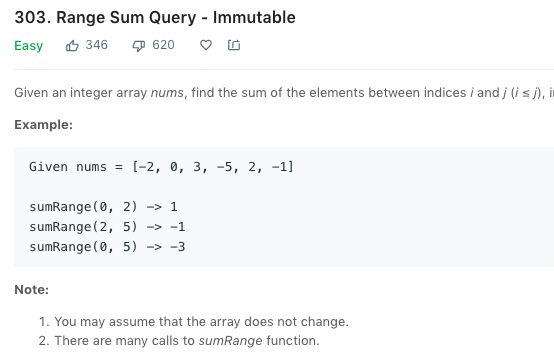``````public class NumArray {
private SegementTree segementTree;

public NumArray(int[] nums) {
segementTree = new SegementTree(nums);
}

public int sumRange(int i, int j) {
if (segementTree == null){
return 0;
}
return segementTree.query(i, j);
}

public static void main(String[] args) {
int[] nums = {-2, 0, 3, -5, 2, -1};
NumArray numArray = new NumArray(nums);
// System.out.println(numArray.sumRange(0, 2));
}
}
``````

#### Update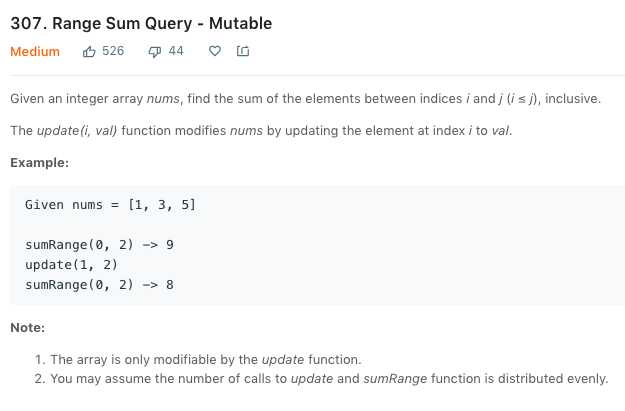``````
public void set(int index, E e) {
if (index < 0 || index >= data.length) {
throw new IllegalArgumentException("index is illgel!!!");
}
data[index] = e;
set(0, 0, data.length - 1, index, e);
}

private void set(int treeIndex, int l, int r, int index, E e) {
if (l == r){
tree[treeIndex] = e;
return;
}
int mid = l + (r - l)/2;
int left = leftChild(index);
int right = rightChild(index);
if (index <= mid){
set(left, l, mid, index, e);
}else if (index > mid){
set(right, mid+1, right, index, e);
}
tree[treeIndex] = merger.merger(tree[left], tree[right]);
}

``````

#### 二维线段树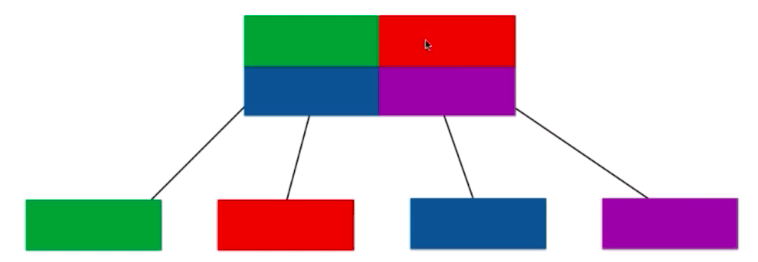# 字典树Trie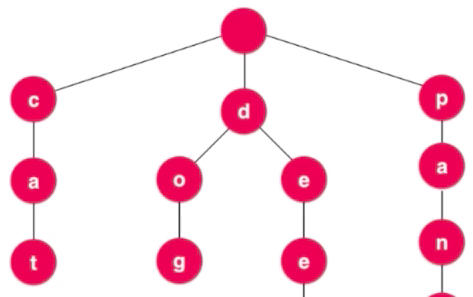#### Create

``````public class Trie {
private class Node {
public boolean isWord;
public TreeMap<Character, Node> next;

public Node(boolean isWord) {
this.isWord = isWord;
next = new TreeMap<>();
}

public Node() {
this(false);
}
}

private Node root;
private int size;

public Trie() {
root = new Node();
this.size = 0;
}

``````

``````    public void add(String word) {
Node cur = root;
for (int i = 0; i < word.length(); i++) {
char c = word.charAt(i);
if (cur.next.get(c) == null) {
cur.next.put(c, new Node());
}
cur = cur.next.get(c);
}
if (!cur.isWord) {
cur.isWord = true;
size++;
}
}

``````

#### Selection

``````    public boolean contains(String word) {
Node cur = root;
for (int i = 0; i < word.length(); i++) {
char c = word.charAt(i);
if (cur.next.get(c) != null){
cur = cur.next.get(c);
}else {
return false;
}
}
return cur.isWord;
}
``````

#### 前缀搜索

``````
public boolean isPrefix(String word){
Node cur = root;
for (int i = 0; i < word.length(); i++) {
char c = word.charAt(i);
if (cur.next.get(c) == null){
return false;
}
cur = cur.next.get(c);
}
return true;
}

``````

#### Leecode208

Leecode里面有一道这样的题目，就是实现字典树：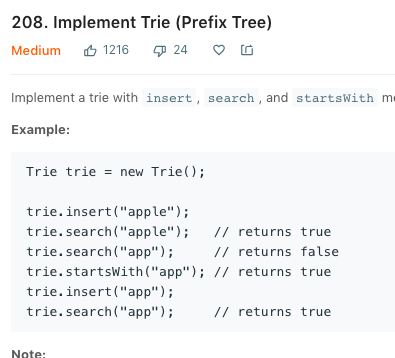#### Leecode211``````
private boolean match(Node node, String word, int index) {
if (index == word.length()) {
return node.isWord;
}
char c = word.charAt(index);
if (c != '.') {
if (node.next.get(c) == null) {
return false;
} else {
return match(node.next.get(c), word, index + 1);
}
} else {
for (char key : node.next.keySet()) {
if (match(node.next.get(key), word, index + 1)) {
return true;
}
}
return false;
}
}

``````

#### Trie作为map使用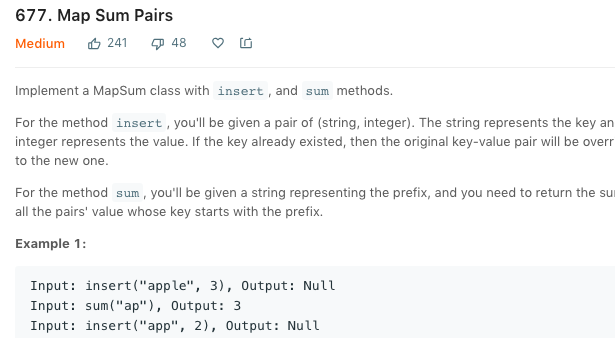``````public int sum(String prefix) {
Node cur = root;
for (int i = 0; i < prefix.length(); i++) {
char c = prefix.charAt(i);
if (cur.next.get(c) == null){
return 0;
}
cur = cur.next.get(c);
}
return sum(cur);
}

private int sum(Node cur){
int res = cur.value;
for (char key : cur.next.keySet()){
res += sum(cur.next.get(key));
}
return res;
}
}
``````

# AVL树

#### 添加操作

1.插入的元素是在不平衡节点的左侧的左侧。也就是一直向左侧插入元素。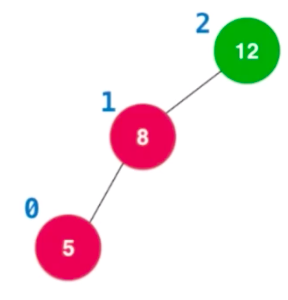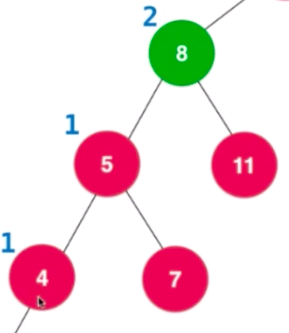##### 右旋转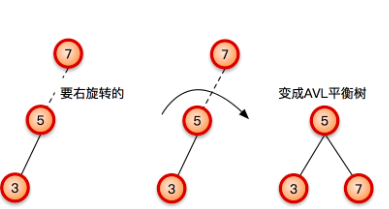5对应的右节点接到7的左节点上，旋转完之后还需要更改高度，先更改子树的高度再更改节点高度。

2.插入的元素是右子树的右子树，也就是一直向右侧插入元素。

##### 左旋转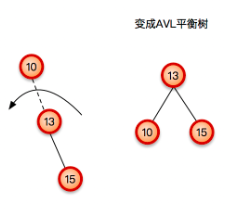3.如果是往左子树的右子树插入了导致不平衡，那么一次旋转是不可以的了。这种情况下只能回到我们之前解决过的情况处理，可以通过先把当前节点的左节点进行左旋转变成情况一，再对当前节点进行右旋转即可。所以就是先对左子树进行左旋转，再对当前节点进行右旋转。
4.如果是往右子树的左节点添加导致了不平衡，那么就需要先右旋转再进行左旋转即可。和上面的情况其实是两两对应的。

``````    public Node add(Node node, K key, V value) {
if (node == null) {
size++;
return new Node(key, value);
}
if (key.compareTo(node.key) > 0) {
} else if (key.compareTo(node.key) < 0) {
} else {
node.value = value;
}
node.height = 1 + Math.max(getHeight(node.left), getHeight(node.right));
int balanceFactor = getBalanceFactor(node);
if (balanceFactor > 1 && getBalanceFactor(node.left) >= 0) {
return rightRotate(node);
}
if (balanceFactor < -1 && getBalanceFactor(node.right) <= 0) {
return leftRotate(node);
}
if (balanceFactor > 1 && getBalanceFactor(node.left) < 0) {
node.left = leftRotate(node.left);
return rightRotate(node);
}
if (balanceFactor < -1 && getBalanceFactor(node.right) > 0) {
node.right = rightRotate(node.right);
return leftRotate(node);
}
return node;
}
``````

# 红黑树

#### 红黑树的特征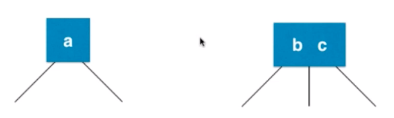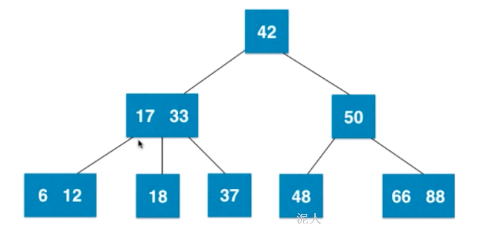#### 那么2-3树是如何维持绝对平衡的？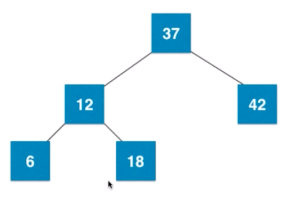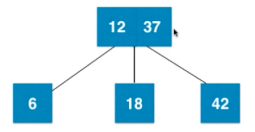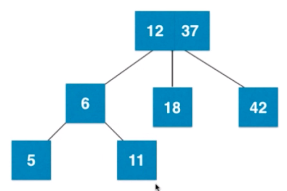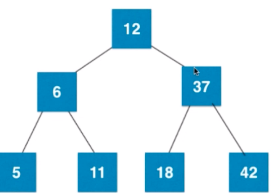### 红黑树的规则

1.每一个节点不是红色就是黑色。
2.根节点总是黑色。
3.如果节点是红色，那么子节点一定是黑色。但是黑色节点下面的子节点可以是红色也可以是黑色。
4.每一个叶子节点，这里的叶子节点不是指有数值的叶子节点，而是指最后的空节点叫做叶子节点，也就是null节点是黑色的。
5.从任意一个节点到叶子节点经过的黑色节点是一样的。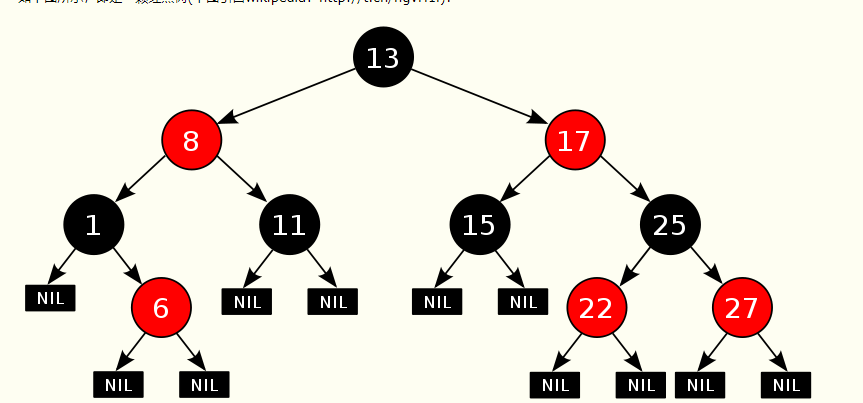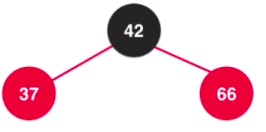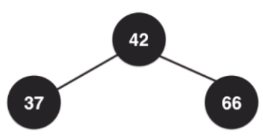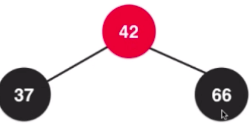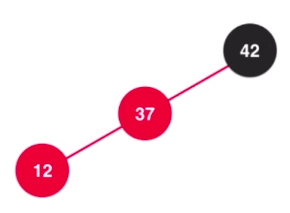#### 添加操作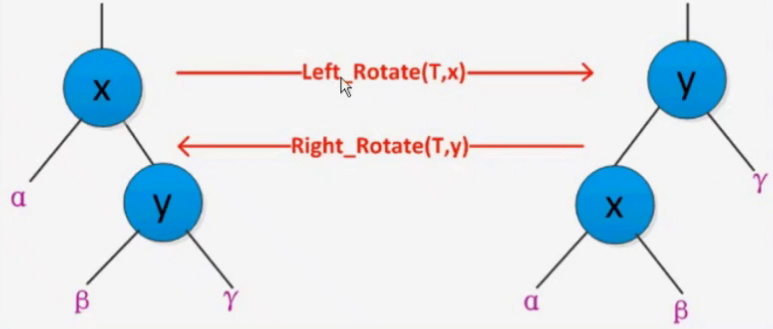①插入的是根节点，那么直接就可以把当前节点变成黑色了，对照规则一，根节点为黑色。同时在2-3树中黑色代表单个节点，这是自然的了。
②父节点是黑色，这种情况下是没有违反任何规则，完美度过。
③当父节点是红色，叔叔节点也是红色的时候，就需要处理了，添加的节点本身就是红色，父亲又是红色，这就违反了性质4。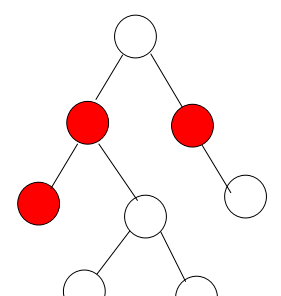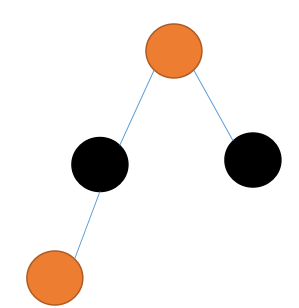④当父节点是红色，叔叔节点为黑色，插入的节点是父节点的左孩子。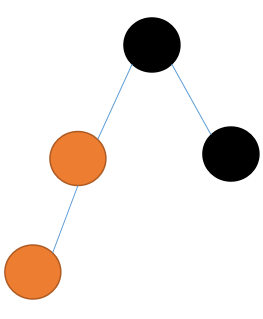⑤当父节点是红色色，叔叔节点是黑色，插入为右孩子。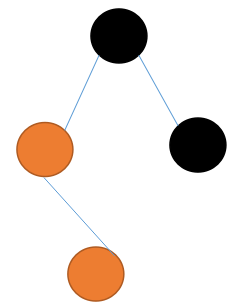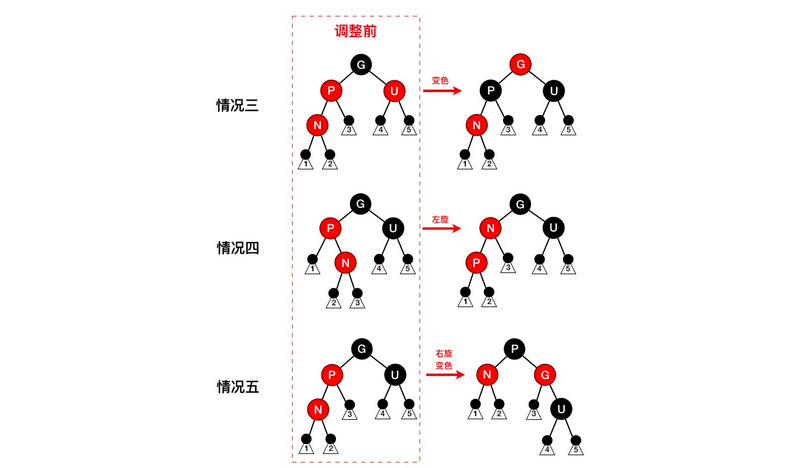#### 删除操作

①删除的就是本身的根节点，而且根节点左右子树是空的。直接删就好了，没有上面好讲的。
②如果删除的节点是红色的，那么它的父亲节点就一定是黑色的。因为是红色的，直接拿孩子节点补充就好了，因为是没有影响的。
③如果删除节点是黑色的，而且兄弟节点是红色的，兄弟节点的孩子节点是黑色，删除节点的父亲节点也是黑色的。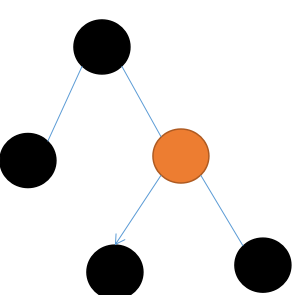④如果删除节点是黑色，父节点和兄弟节点以及兄弟节点的孩子都是黑色。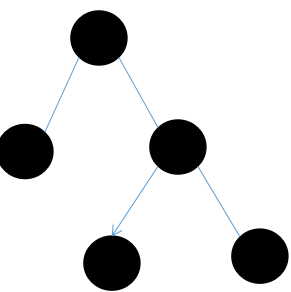⑤删除的节点为黑色，父亲节点为红色，兄弟节点和兄弟节点的孩子为黑色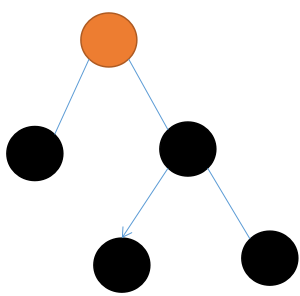⑥删除的节点是黑色，兄弟节点也是黑色，兄弟孩子的左节点是红色，兄弟节点的右子树为黑色，父亲节点随便颜色。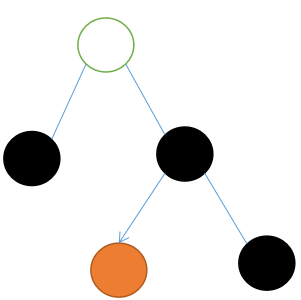⑦删除的节点是黑色，兄弟节点也是黑色，兄弟节点的右孩子为红色，父亲节点和兄弟节点左孩子随便颜色。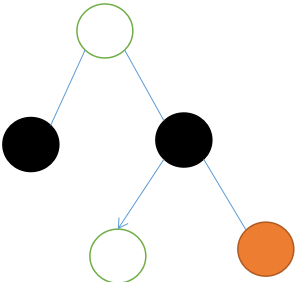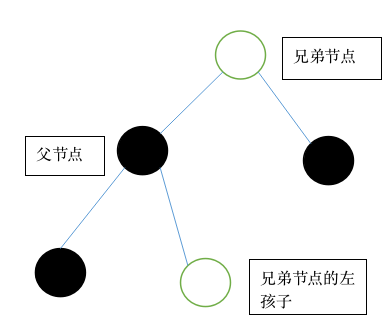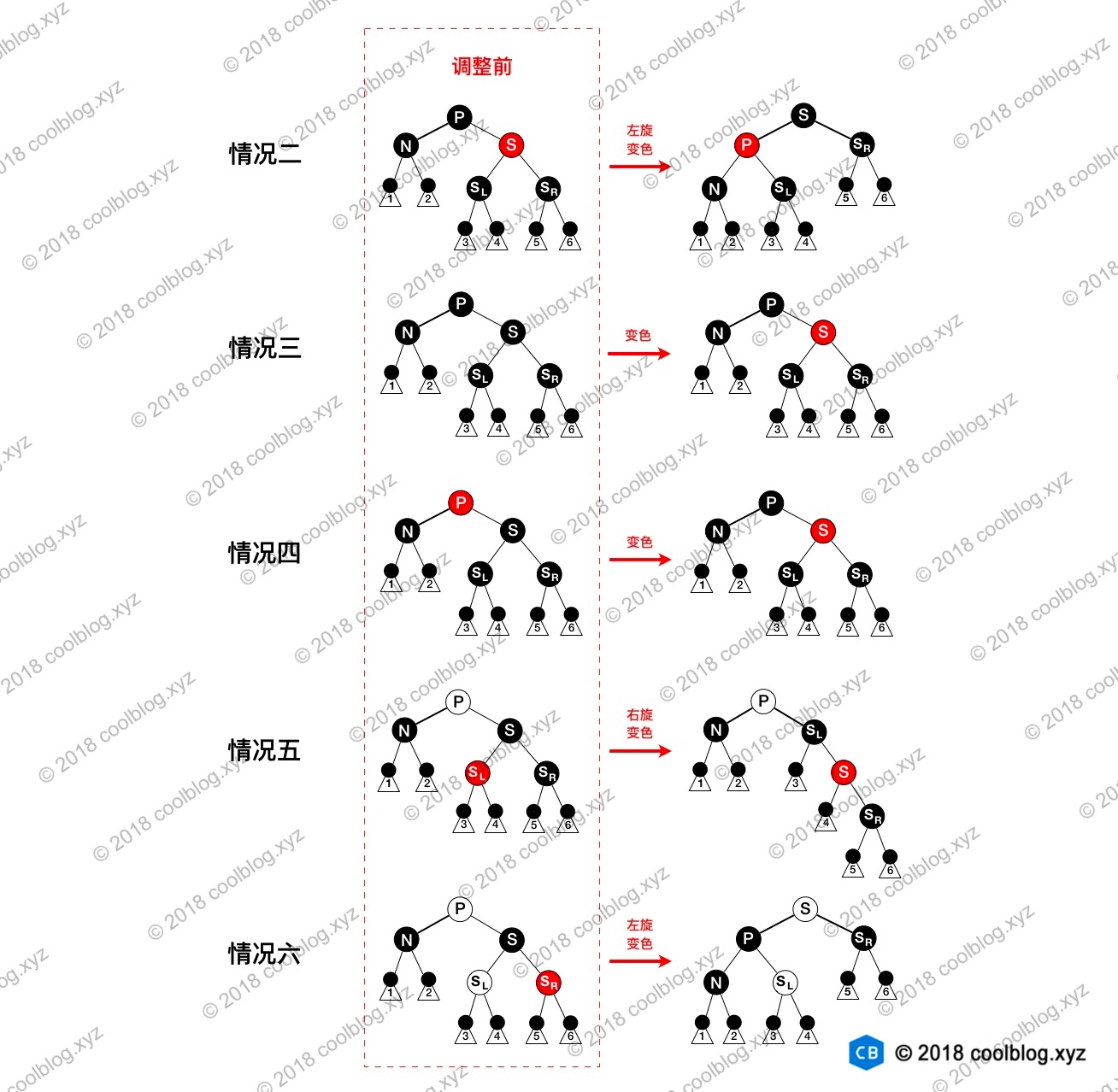#### 红黑树的实现

``````    private class Node<T extends Comparable<T>> {
public boolean color;
public T key;
Node<T> left;
Node<T> right;
Node<T> parent;

public Node(T key, boolean color, Node<T> parent, Node<T> left, Node<T> right) {
this.key = key;
this.color = color;
this.parent = parent;
this.left = left;
this.right = right;
}

public T getKey() {
return key;
}

@Override
public String toString() {
String s = "" + key + (this.color == RED ? "R" : "B");
if (this.left != null) {
s += ("  " + left.key);
}
if (this.right != null) {
s += ("  " + right.key);
}
return s;
}
}

``````

``````    private void insertFixUp(Node<T> node) {
Node<T> parent, grandParent;
while ((parent = parentOf(node)) != null && isRed(parent)) {
grandParent = parentOf(parent);
if (parent == grandParent.left) {
//third condition,the uncle is red
Node<T> uncle = grandParent.right;
if (uncle != null && isRed(uncle)) {
setBlack(uncle);
setBlack(parent);
setRed(grandParent);
node = grandParent;
continue;
}
//fifth condition,the uncle is black and left child of the current node
if (parent.right == node) {
Node<T> temp;
leftRotate(parent);
temp = parent;
parent = node;
node = temp;
}
//fourth condiction,same as above but the right child of the current node.
setBlack(parent);
setRed(grandParent);
rightRotate(grandParent);
} else {
//third condition,the uncle is red.
Node<T> uncle = grandParent.left;
if (uncle != null && isRed(uncle)) {
setBlack(uncle);
setBlack(parent);
setRed(grandParent);
node = grandParent;
continue;
}
//fifth condition,the uncle is black,left child
if (parent.left == node) {
Node<T> temp;
rightRotate(parent);
temp = parent;
parent = node;
node = temp;
}
//fourth condition,the uncle is black,right child
setBlack(parent);
setRed(grandParent);
leftRotate(grandParent);
}
}
setBlack(root);
}

``````

``````    private void removeFixUp(Node<T> node, Node<T> par) {
Node<T> uncle;
Node<T> parent;
parent = node == null ? par : node.parent;
while ((node == null || isBlack(node)) && node != root) {
if (parent.left == node) {
uncle = parent.right;
//the uncle is red, condition three
if (isRed(uncle)) {
setBlack(uncle);
setRed(parent);
leftRotate(parent);
uncle = parent.right;
}
//the uncle and his child are all black
if ((uncle.left == null || isBlack(uncle.left)) &&
(uncle.right == null || isBlack(uncle.right))) {
setRed(uncle);
node = parent;
parent = parentOf(node);
} else {
//the uncle is black and red of his child on the left
if (uncle.right == null || isBlack(uncle.right)) {
setBlack(uncle.left);
setRed(uncle);
rightRotate(uncle);
uncle = parent.right;
}
setColor(uncle, colorOf(parent));
setBlack(parent);
setBlack(uncle.right);
leftRotate(parent);
node = this.root;
break;
}
} else {
uncle = parent.left;
if (isRed(uncle)) {
setBlack(uncle);
setRed(parent);
rightRotate(parent);
uncle = parent.left;
}
if ((uncle.left == null || isBlack(uncle.left)) &&
(uncle.right == null || isBlack(uncle.right))) {
setRed(uncle);
node = parent;
parent = parentOf(node);
} else {
if (uncle.left == null || isBlack(uncle.left)) {
setBlack(uncle.right);
setRed(uncle);
leftRotate(uncle);
uncle = parent.left;
}
setColor(uncle, colorOf(parent));
setBlack(parent);
setBlack(uncle.left);
rightRotate(parent);
node = this.root;
break;
}
}
}
if (node != null) {
setBlack(node);
}
}

``````

+ 关注